# Electronics and Communication Engineering - Exam Questions Papers

36.

In the following circuit , the comparator output in log: "I" if V1 > V2 and is logic "0" otherwise. The D/A conversion is done as per the relations VDAC =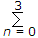2n-2 Volts, where b3 (MSB), b2, b1 and b0 (LSB) are the counter outputs. The counter starts from the clear state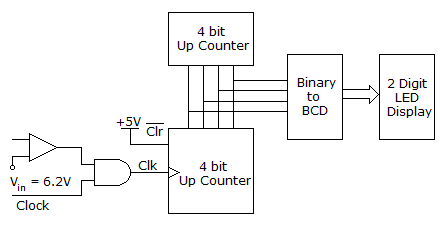The magnitude of the error between VDAC and Vin at steady state in volts is

 A. 0.2 B. 0.3 C. 0.5 D. 1

Answer: Option C

Explanation:

Magnitude error = 6.5 - 6.2 = 0.3 .

37.

The signal flow graph of a system is shown below: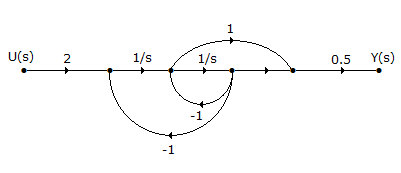The state variable representation of the system can be

 A.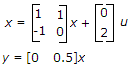B.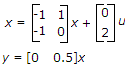C.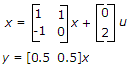D.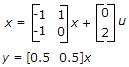Answer: Option D

Explanation:

Let us take two state variable as shown x1 and x2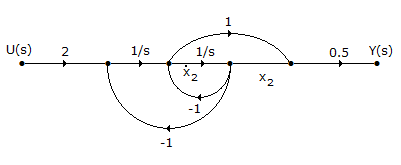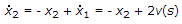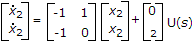38.

Consider the resonant circuit as shown below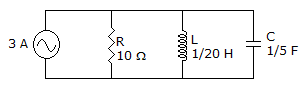The circuit is scaled modified so that Zmax 104 and ωo = 108 Radians. Find the required element values

 A. R = 104 L = 5 μH C = 20 pF B. 1000 L = 50 μH C = 200 pF C. 10 K L = 50 μH C =20 pF D. None of these

Answer: Option C

Explanation:

Impedance scaling factor kx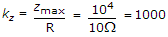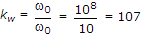As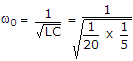= 10 Radians

New value for elements are

R' = kzR = 1000 x 10 = 10 kΩ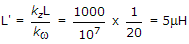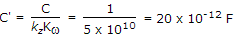.

39.

Consider the z-transform X(z) = 5z2 + 4z-1 + 3; 0 < |z| < ∞ The inverse transform x[n] is

 A. 5δ[n + 2] + 3δ[n] + 4δ[n - 1] B. 5δ[n - 2] + 3δ[n] + 4δ[n + 1] C. 5u[n + 2] + 3u[n] + 4u[n - 1] D. 5u[n - 2] + 3u[n] + 4u[n + 1]

Answer: Option A

Explanation:

X(z) = 5z2 + 4z-1 + 3 =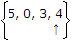.

40.

Find equivalent capacitance if each capacitance is 2 F.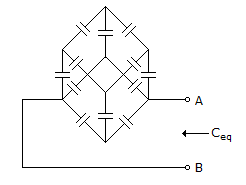A. 5/12 F B. 12/5 F C. 6/5 F D. 5/6 F

Answer: Option B

Explanation:

For capacitor take inverse of value of capacitance and then apply procedure same as in resistance case.

After getting the final answer take inverse of result to get Ceq

Ceq =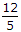F

In Cuboids case,

For resistance Req =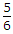R Ω with each R = 1

For inductance Leq =L H with each L = 1 H

For capacitance Ceq =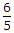CF with each C = 1 F.

#### Current Affairs 2021

Interview Questions and Answers